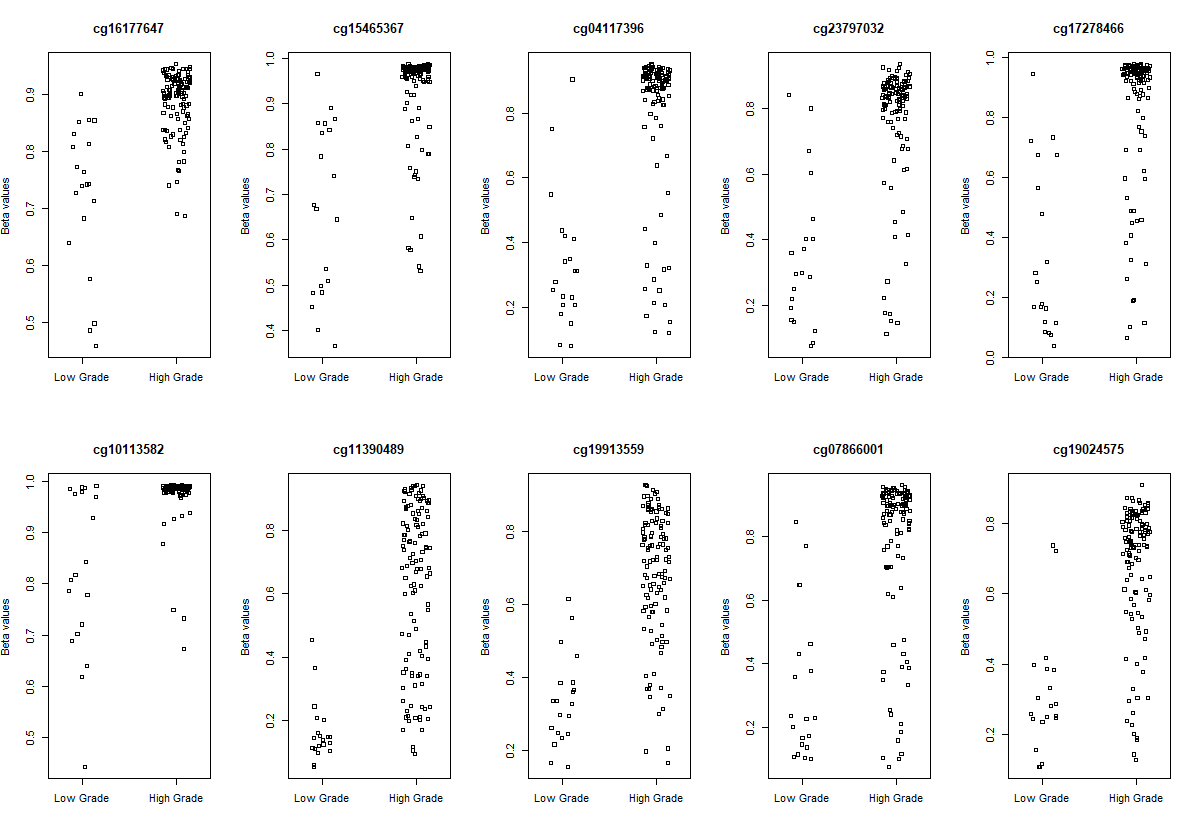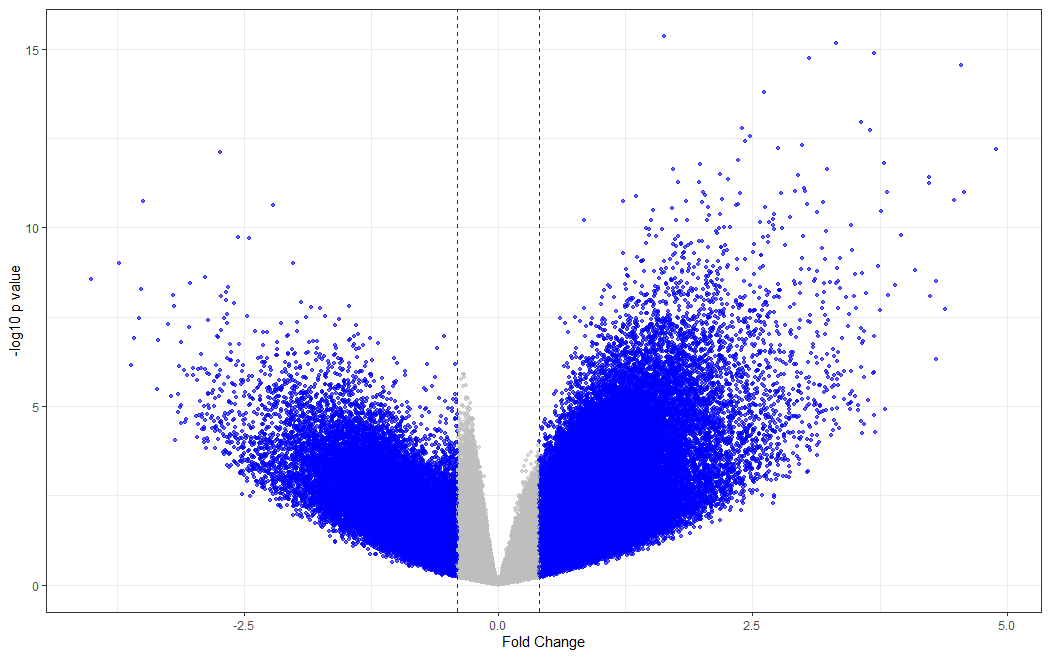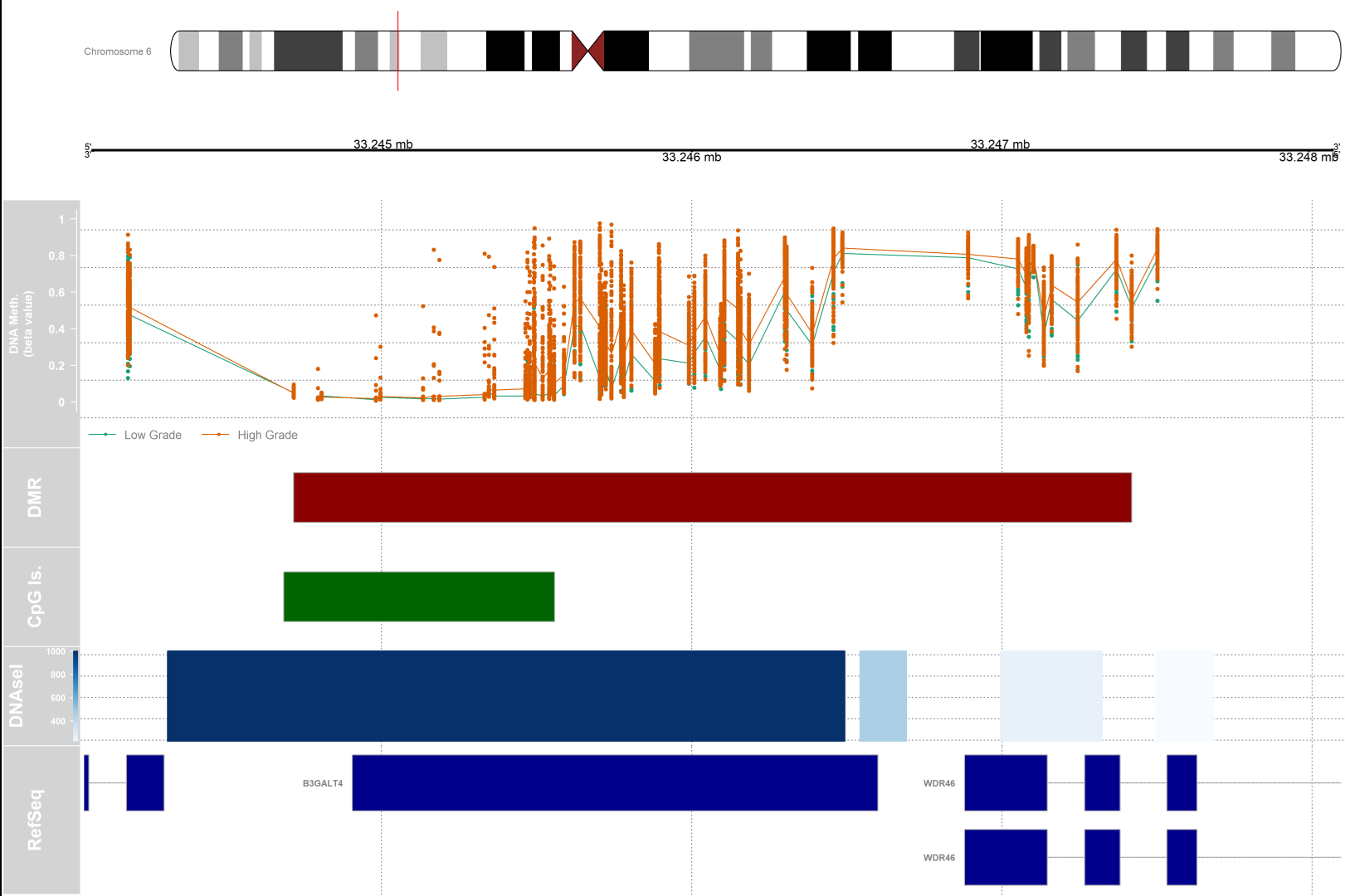Biostar Test Site

This is site is used for testing only. Visit: https://www.biostars.org to ask a question.

Tutorial:Methylation Analysis Tutorial in R_part1
0
17
Entering edit mode
8 months ago
Hamid Ghaedi ★ 1.4k

The code and approaches that I share here are those I am using to analyze TCGA methylation data. At the bottom of the page, you can find references used to make this tutorial. If you are coming from a computer background, please bear with a geneticist tried to write code :). Your feedback worth a lot to me.

The three main analysis of methylation data are covered:

1-differential methylation analysis

2- differential variability analysis

3- integrative analysis

For codes and files you can check my GitHub. For this post, I used publicly available data from TCGA bladder cancer (TCGA-BLCA) for methylation and gene expression analysis.

## Workspace preparation

#______________loading packages__________________#
library(TCGAbiolinks)
library(IlluminaHumanMethylation450kanno.ilmn12.hg19)
library(IlluminaHumanMethylation450kmanifest)
library(minfi)
library(limma)
library(missMethyl)
library(DMRcate)
library(Gviz)
library(ggplot2)
library(RColorBrewer)
library(edgeR)

#increase memory size
memory.limit(size = 28000) # do this on your own risk!


## Data download and preparation

#_________ DNA methylation Download____________#
# DNA methylation aligned to hg19
query_met <- GDCquery(project= "TCGA-BLCA",
data.category = "DNA methylation",
platform = "Illumina Human Methylation 450",
legacy = TRUE)
GDCdownload(query_met)
#putting files togathers
data.met <- GDCprepare(query_met)
#saving the met object
saveRDS(object = data.met,
file = "data.met.RDS",
compress = FALSE)
# loading saved session: Once you saved your data, you can load it into your environment:
data.met = readRDS(file = "data.met.RDS")
# met matrix
met <- as.data.frame(SummarizedExperiment::assay(data.met))
# clinical data
clinical <- data.frame(data.met@colData)

#___________inspecting methylation data_______________#

# get the 450k annotation data
ann450k <- getAnnotation(IlluminaHumanMethylation450kanno.ilmn12.hg19)

## remove probes with NA
probe.na <- rowSums(is.na(met))

table(probe.na == 0)
#FALSE   TRUE
#103553 382024
# chose those has not NA values in rows
probe <- probe.na[probe.na == 0]
met <- met[row.names(met) %in% names(probe), ]

## remove probes that match to chromosome  X and Y
keep <- !(row.names(met) %in% ann450k$Name[ann450k$chr %in% c("chrX","chrY")])
table(keep)
met <- met[keep, ]
rm(keep) # remove no further needed probes.

## remove SNPs overlapped probe
table (is.na(ann450k$Probe_rs)) # probes without snp no.snp.probe <- ann450k$Name[is.na(ann450k$Probe_rs)] snp.probe <- ann450k[!is.na(ann450k$Probe_rs), ]
#snps with maf <= 0.05
snp5.probe <- snp.probe$Name[snp.probe$Probe_maf <= 0.05]

# filter met
met <- met[row.names(met) %in% c(no.snp.probe, snp5.probe), ]

#remove no-further needed dataset
rm(no.snp.probe, probe, probe.na, snp.probe, snp5.probe)

## Removing probes that have been demonstrated to map to multiple places in the genome.
# list adapted from https://www.tandfonline.com/doi/full/10.4161/epi.23470

crs.reac <- read.csv("cross_reactive_probe.chen2013.csv")
crs.reac <- crs.reac$TargetID[-1] # filtre met met <- met[ -which(row.names(met) %in% crs.reac), ] bval <- met ## converting beta values to m_values ## m = log2(beta/1-beta) mval <- t(apply(met, 1, function(x) log2(x/(1-x)))) #______________saving/loading_____________________# # save data sets #saveRDS(mval, file = "mval.RDS", compress = FALSE) #saveRDS (bval, file = "bval.RDS", compress = FALSE) #mval <- readRDS("mval.RDS") #bval <- readRDS("bval.RDS")  ## Differential methylation analysis ## Different methylated CpGs (DMC) or Probe-wise differential methylation analysis Here we are interested to find probes that are differentially methylated between low-grade and high-grade bladder cancer. In the clinical dataset, there is column paper_Histologic.grade that provides data on tumor grade. Also as one can see, most low-grade bladder cancer samples belong to the luminal papillary subtype. I have to make it clear that for assigning "low-grade" or "high-grade" to a sample I relied on the clinical data published by A Gordon Robertson, however, it is acknowledged that those samples in the Ta stage should be considered as "low-grade", samples with T1 and upper stages are "high-grade". By the way, this would not affect a bioinformatic analysis! table(clinical$paper_Histologic.grade, clinical$paper_mRNA.cluster) # Basal_squamous Luminal Luminal_infiltrated Luminal_papillary ND Neuronal # High Grade 143 28 79 120 4 20 # Low Grade 1 0 0 20 0 0 # ND 0 0 1 2 0 0 # filtering and grouping clinical <- clinical[, c("barcode", "paper_Histologic.grade", "paper_mRNA.cluster")] clinical <- na.omit(clinical) clinical <- clinical[-which(clinical$paper_mRNA.cluster == "ND"), ]
clinical <- clinical[-which(clinical$paper_Histologic.grade == "ND"), ] clinical <- clinical[which(clinical$paper_mRNA.cluster == "Luminal_papillary"), ]
barcode <- clinical$barcode # removing samples from meth matrixes bval <- bval[, colnames(bval) %in% barcode] mval <- mval[, colnames(mval) %in% barcode] # Making sure about samples in clinical and matrixes and their order table(colnames(mval) %in% row.names(clinical)) table(colnames(bval) %in% row.names(clinical)) # all(row.names(clinical) == colnames(bval)) all(row.names(clinical) == colnames(mval)) #Making grouping variable clinical$paper_Histologic.grade <- as.factor(clinical$paper_Histologic.grade) #levels(clinical$paper_Histologic.grade)
clinical$paper_Histologic.grade <- relevel(clinical$paper_Histologic.grade, ref = "Low Grade")

#_____________ DMC analysis________________#
design <- model.matrix(~ paper_Histologic.grade, data = clinical)
# fit the linear model
fit <- lmFit(mval, design)
fit2 <- eBayes(fit)

# extracting significantly methylated probes
deff.meth = topTable(fit2, coef=ncol(design), sort.by="p",number = nrow(mval), adjust.method = "BY")
# Visualization
# plot the top 10 most significantly differentially methylated CpGs
par(mfrow=c(2,5))
sapply(rownames(deff.meth)[1:10], function(cpg){
plotCpg(bval, cpg=cpg, pheno=clinical$paper_Histologic.grade, ylab = "Beta values") })# making a volcano plot #making dataset dat <- data.frame(foldchange = fit[["coefficients"]][,2], logPvalue = -log10(fit2[["p.value"]][,2])) dat$threshold <- as.factor(abs(dat$foldchange) < 0.4) #Visualization cols <- c("TRUE" = "grey", "FALSE" = "blue") ggplot(data=dat, aes(x=foldchange, y = logPvalue, color=threshold)) + geom_point(alpha=.6, size=1.2) + scale_colour_manual(values = cols) + geom_vline(xintercept = 0.4, colour="#990000", linetype="dashed") + geom_vline(xintercept = - 0.4, colour="#990000", linetype="dashed") + theme(legend.position="none") + xlab("Fold Change") + ylab("-log10 p value") + theme_bw() + theme(legend.position = "none")## Differentially methylated regions (DMRs) analysis # setting some annotation myAnnotation <- cpg.annotate(object = mval, datatype = "array", what = "M", analysis.type = "differential", design = design, contrasts = FALSE, coef = "paper_Histologic.gradeHigh Grade", arraytype = "450K", fdr = 0.001) str(myAnnotation) # DMR analysis DMRs <- dmrcate(myAnnotation, lambda=1000, C=2) results.ranges <- extractRanges(DMRs) results.ranges # visualization dmr.table <- data.frame(results.ranges) # setting up a variable for grouping and color pal <- brewer.pal(8,"Dark2") groups <- pal[1:length(unique(clinical$paper_Histologic.grade))]
names(groups) <- levels(factor(clinical$paper_Histologic.grade)) #setting up the genomic region gen <- "hg19" # the index of the DMR that we will plot dmrIndex <- 2 # coordinates are stored under results.ranges[dmrIndex] chrom <- as.character(seqnames(results.ranges[dmrIndex])) start <- as.numeric(start(results.ranges[dmrIndex])) end <- as.numeric(end(results.ranges[dmrIndex])) # add 25% extra space to plot minbase <- start - (0.25*(end-start)) maxbase <- end + (0.25*(end-start)) # defining CpG islands track # download cpgislands for chromosome number 6 from ucsc chr6.cpg <- read.csv("chr6-cpg.csv") islandData <- GRanges(seqnames=Rle(chr6.cpg[,1]), ranges=IRanges(start=chr6.cpg[,2], end=chr6.cpg[,3]), strand=Rle(strand(rep("*",nrow(chr6.cpg))))) # DNAseI hypersensitive sites track #downloaded from ucsc chr6.dnase <- read.csv("chr6-dnase.csv") dnaseData <- GRanges(seqnames=chr6.dnase[,1], ranges=IRanges(start=chr6.dnase[,2], end=chr6.dnase[,3]), strand=Rle(rep("*",nrow(chr6.dnase))), data=chr6.dnase[,5]) #Setting up the ideogram, genome, and RefSeq tracks iTrack <- IdeogramTrack(genome = gen, chromosome = chrom, name=paste0(chrom)) gTrack <- GenomeAxisTrack(col="black", cex=1, name="", fontcolor="black") rTrack <- UcscTrack(genome=gen, chromosome=chrom, track="NCBI RefSeq", from=minbase, to=maxbase, trackType="GeneRegionTrack", rstarts="exonStarts", rends="exonEnds", gene="name", symbol="name2", transcript="name", strand="strand", fill="darkblue",stacking="squish", name="RefSeq", showId=TRUE, geneSymbol=TRUE) #Ensure that the methylation data is ordered by chromosome and base position. ann450kOrd <- ann450k[order(ann450k$chr,ann450k$pos),] bvalOrd <- bval[match(ann450kOrd$Name,rownames(bval)),]

#Create the data tracks:
# create genomic ranges object from methylation data
cpgData <- GRanges(seqnames=Rle(ann450kOrd$chr), ranges=IRanges(start=ann450kOrd$pos, end=ann450kOrd$pos), strand=Rle(rep("*",nrow(ann450kOrd))), betas=bvalOrd) # methylation data track methTrack <- DataTrack(range=cpgData, groups=clinical$paper_Histologic.grade, # change this if your groups are diffrent
genome = gen,
chromosome=chrom,
ylim=c(-0.05,1.05),
col=pal,
type=c("a","p"),
name="DNA Meth.\n(beta value)",
background.panel="white",
legend=TRUE,
cex.title=0.8,
cex.axis=0.8,
cex.legend=0.8)

# CpG island track
islandTrack <- AnnotationTrack(range=islandData, genome=gen, name="CpG Is.",
chromosome=chrom,fill="darkgreen")

# DNaseI hypersensitive site data track
dnaseTrack <- DataTrack(range=dnaseData, genome=gen, name="DNAseI",
type="gradient", chromosome=chrom)

# DMR position data track
dmrTrack <- AnnotationTrack(start=start, end=end, genome=gen, name="DMR",
chromosome=chrom,fill="darkred")

# Set up the tracklist and indicate the relative sizes of the different tracks.
# Finally, draw the plot using the plotTracks function
tracks <- list(iTrack, gTrack, methTrack, dmrTrack, islandTrack, dnaseTrack,
rTrack)
sizes <- c(2,2,5,2,2,2,3) # set up the relative sizes of the tracks
# to save figure and scaling graphic device
tiff( filename = "dmr.tiff", width = 15, height = 10, units = "in", res = 400)
plotTracks(tracks, from=minbase, to=maxbase, showTitle=TRUE, add53=TRUE,
add35=TRUE, grid=TRUE, lty.grid=3, sizes = sizes, length(tracks))
dev.off()References

Tutorial cancer methylation R • 3.2k views
ADD COMMENT
1
Entering edit mode

thank you so much it was really useful to me

ADD REPLY

Login before adding your answer.

Traffic: 173 users visited in the last hour
Help About
FAQ
Access RSS
API
Stats

Use of this site constitutes acceptance of our User Agreement and Privacy Policy.